# Setup and use onlineforecast models

## Intro

This vignette explains how to setup and use an onlineforecast model. This takes offset in the example of building heat load forecasting and assumes that the data is setup correctly, as explained in setup-data vignette. The R code is available here. More information on onlineforecasting.org.

# Load the package
library(onlineforecast)
# Set the data in D to simplify notation
D <- Dbuilding

## Score period

Set the scoreperiod as a logical vector with same length as t. It controls which points are included in score calculations in functions for optimization etc. It must be set.

Use it to exclude a burn-in period of one week:

# Print the first time point
D$t ##  "2010-12-15 01:00:00 UTC" # Set the score period D$scoreperiod <- in_range("2010-12-22", D$t) # Plot to see it plot(D$t, D$scoreperiod, xlab="Time", ylab="Scoreperiod")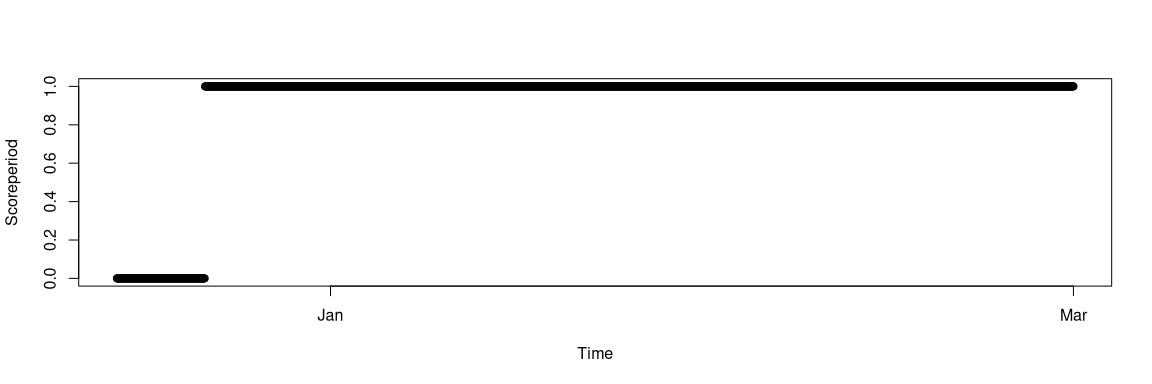Other periods, which should be excluded from score calculations, can simply also be set to FALSE. E.g.: # Exclude other points example scoreperiod2 <- D$scoreperiod
scoreperiod2[in_range("2010-12-30",D$t,"2011-01-02")] <- FALSE would exclude the days around new year (must of course be set in D$scoreperiod, not in scoreperiod2 to have an effect).

## Setting up a model

A simple onlineforecast model can be setup by:

# Generate new object (R6 class)
model <- forecastmodel$new() # Set the model output model$output = "heatload"
# Inputs (transformation step)
model$add_inputs(Ta = "Ta", mu = "one()") # Regression step parameters model$add_regprm("rls_prm(lambda=0.9)")
# Optimization bounds for parameters
model$add_prmbounds(lambda = c(0.9, 0.99, 0.9999)) # Set the horizons for which the model will be fitted model$kseq <- 1:36

### Steps in setting up a model

Let’s go through the steps of setting up the model.

First a new forecastmodel object is generated and the model output is set (per default it is "y"):

# Generate new object
model <- forecastmodel$new() # Set the model output model$output = "heatload"

The output is simply the variable name from D we want to forecast.

The model inputs are defined by:

# Inputs (transformation step)
model$add_inputs(Ta = "Ta", mu = "one()") So this is really where the structure of the model is specified. The inputs are given a name (Ta and mu), which each are set as an R expression (given as a string). The expressions defines the transformation step: they will each be evaluated in an environment with a given data.list. This means that the variables from the data can be used in the expressions (e.g. Ta is in D) - below in Input transformations we will detail this evaluation. Next step for setting up the model is to set the parameters for the regression step by providing an expression, which returns the regression parameter values. In the present case we will use the Recursive Least Squares (RLS) when regressing, and we need to set the forgetting factor lambda by: # Regression step parameters model$add_regprm("rls_prm(lambda=0.9)")

The expression is just of a function, which returns a list - in this case with the value of lambda (see onlineforecasting). The result of it begin evaluated is kept in:

# The evaluation happens with
eval(parse(text="rls_prm(lambda=0.9)"))
##     $lambda ##  0.9 # and the result is stored in model$regprm
##     $lambda ##  0.9 We will tune the parameters, for this model it’s only the forgetting factor, so we set the parameter bounds (lower, init, upper) for it by: # Optimization bounds for parameters model$add_prmbounds(lambda = c(0.9, 0.99, 0.9999))

Finally, we set the horizons for which to fit:

# Set the horizons for which the model will be fitted
model$kseq <- 1:36 The horizons to fit for is actually not directly related to the model, but rather the fitting of the model. In principle, it would be more “clean” if the model, data and fit was kept separate, however for recursive fitting this becomes un-feasible. ### Tune the parameters We have set up the model and can now tune the lambda with the rls_optim(), which is a wrapper for the optim() function: # Call the optim() wrapper rls_optim(model, D, kseq = c(3,18)) ## ---------------- ## lambda ## 0.99 ## k3 k18 sum ## 0.831 0.826 1.657 ## ---------------- ## lambda ## 0.991 ## k3 k18 sum ## 0.831 0.827 1.658 ## ---------------- ## lambda ## 0.989 ## k3 k18 sum ## 0.831 0.826 1.657 ## ...output cropped Note, how it only calculated a score for the 3 and 18 steps horizons - since we gave it kseq as an argument, which then overwrites model$kseq for the optimization only. The parameters could be optimized separately for each horizon, for example it is often such that for the first horizons a very low forgetting factor is optimal (e.g. 0.9). Currently, however, the parameters can only be optimized together. By optimizing for a short (3 steps) and a long horizon (18 steps), we obtain a balance - using less computations compared to optimizing on all horizons.

The optimization converge and the tuned parameter was inserted:

# Optimized lambda
model$prm ## lambda ## 0.985 Now we can fit with the optimized lambda on the horizons in model$kseq over the entire period:

# Fit for all on entire period in D
fit1 <- rls_fit(modelprm, model, D) ## ---------------- ## lambda ## 0.985 See the summary of the fit: # See the summary of the fit summary(fit1) ## ## Output: heatload ## Inputs: Ta = Ta ## mu = one() ## ## Regression parameters: ## lambda = 0.985 ## ## Scoreperiod: 1656 observations are included. ## ## RLS coeffients summary stats (cannot be used for significance tests): ## mean sd min max ## Ta -0.14 0.044 -0.26 0.19 ## mu 5.20 0.280 4.50 6.80 ## ## RMSE: ## k1 k2 k3 k4 k5 k6 k7 k8 k9 k10 k11 k12 k13 k14 k15 k16 ## 0.82 0.83 0.83 0.83 0.83 0.84 0.84 0.84 0.84 0.84 0.83 0.83 0.83 0.83 0.83 0.83 ## k17 k18 k19 k20 k21 k22 k23 k24 k25 k26 k27 k28 k29 k30 k31 k32 ## 0.83 0.83 0.83 0.82 0.83 0.83 0.83 0.84 0.85 0.86 0.86 0.86 0.86 0.86 0.86 0.86 ## k33 k34 k35 k36 ## 0.86 0.86 0.86 0.86 See ?summary.rls_fit for details. Plot the forecasts (Yhat adheres to the forecast matrix format and in plot_ts() the forecasts are lagged k steps to be aligned with the observations): # Put the forecasts in D DYhat1 <- fit1$Yhat # Plot them for selected horizons plot_ts(D, c("^heatload$|^Y"), kseq = c(1,6,18,36))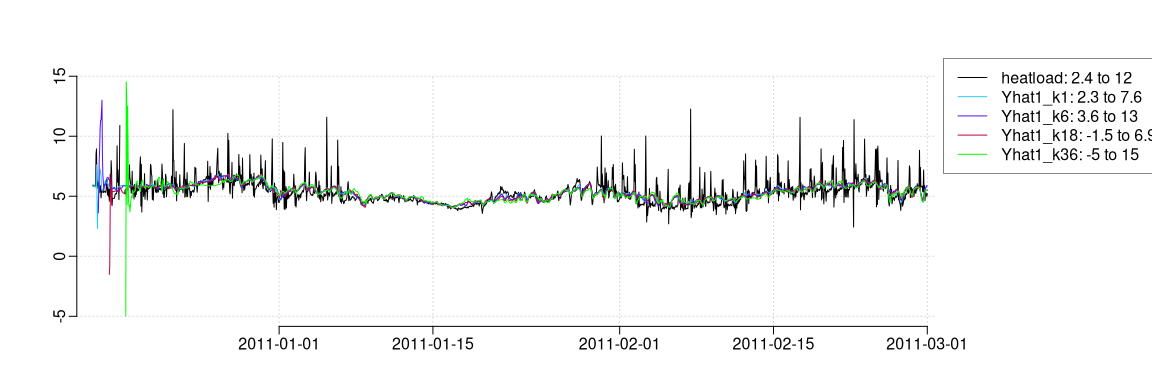We clearly see the burn-in period, where the forecasts vary a lot,

Plot a forecast for a particular time point and forward in time:

# Select a point
i <- 996-48
# and kseq steps ahead
iseq <- i+model$kseq # The observations ahead in time plot(D$t[iseq], D$heatload[iseq], type = "b", xlab = "t", ylab = "y") title(main=pst("Forecast available at ",D$t[i]))
# The forecasts
lines(D$t[iseq], D$Yhat1[i, ], type = "b", col = 2)
legend("topright", c("Observations",pst("Predictions (",min(model$kseq)," to ",max(model$kseq)," steps ahead)")), lty = 1, col = 1:2)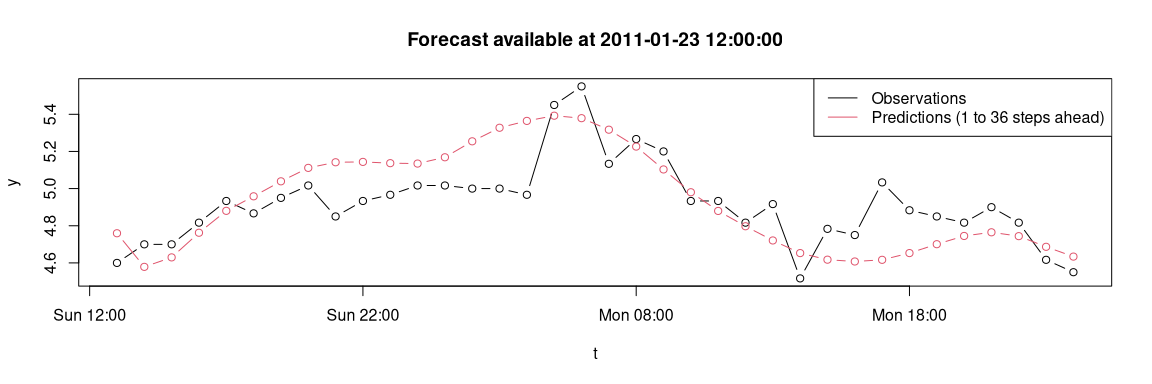## Input transformations

The inputs can be transformations of the variables in the data, i.e. D in this example. The function one() generate a forecast matrix of 1 for the needed horizons. It cannot be called directly:

# This will give error
one()

(the code above was not executed)

however we can see the result of the evaluation by:

# Evaluate input expressions
datatr <- model$transform_data(D) # See what came out summary.default(datatr) ## Length Class Mode ## Ta 36 data.frame list ## mu 36 data.frame list # In particular for the mu = "one()" head(datatr$mu)
##       k1 k2 k3 k4 k5 k6 k7 k8 k9 k10 k11 k12 k13 k14 k15 k16 k17 k18 k19 k20 k21
##     1  1  1  1  1  1  1  1  1  1   1   1   1   1   1   1   1   1   1   1   1   1
##     2  1  1  1  1  1  1  1  1  1   1   1   1   1   1   1   1   1   1   1   1   1
##     3  1  1  1  1  1  1  1  1  1   1   1   1   1   1   1   1   1   1   1   1   1
##     4  1  1  1  1  1  1  1  1  1   1   1   1   1   1   1   1   1   1   1   1   1
##     5  1  1  1  1  1  1  1  1  1   1   1   1   1   1   1   1   1   1   1   1   1
##     6  1  1  1  1  1  1  1  1  1   1   1   1   1   1   1   1   1   1   1   1   1
##       k22 k23 k24 k25 k26 k27 k28 k29 k30 k31 k32 k33 k34 k35 k36
##     1   1   1   1   1   1   1   1   1   1   1   1   1   1   1   1
##     2   1   1   1   1   1   1   1   1   1   1   1   1   1   1   1
##     3   1   1   1   1   1   1   1   1   1   1   1   1   1   1   1
##     4   1   1   1   1   1   1   1   1   1   1   1   1   1   1   1
##     5   1   1   1   1   1   1   1   1   1   1   1   1   1   1   1
##     6   1   1   1   1   1   1   1   1   1   1   1   1   1   1   1

If we wanted to debug we could:

# Set the function to debug (uncomment the line)
#debug(one)
# Run the input transformation now and it will stop in one()
datatr <- model$transform_data(D) # Set to undebug #undebug(one) (the code above was not executed). Let’s extend the model by adding a low-pass filter transformation of the ambient temperature forecasts. We could just update the input by: # Just update the Ta input by model$add_inputs(Ta = "lp(Ta, a1=0.9)")

but let’s just repeat the whole model definition for clarification - including the new transformation:

# Define a new model with low-pass filtering of the Ta input
model <- forecastmodel$new() model$output = "heatload"
model$add_inputs(Ta = "lp(Ta, a1=0.9)", mu = "one()") model$add_regprm("rls_prm(lambda=0.99)")
model$add_prmbounds(Ta__a1 = c(0.5, 0.9, 0.9999), lambda = c(0.9, 0.99, 0.9999)) model$kseq <- c(3,18)

Note how also a new set of parameter bounds were added in add_prmbounds() following a neat little syntax: Ta__a1 indicates that the first appearance of a1 in the Ta input expression, will be changed in the optimization.

We can see the parameter bounds with:

model$prmbounds ## lower init upper ## Ta__a1 0.5 0.90 1 ## lambda 0.9 0.99 1 To inspect the result of low-pass filtering: # Low-pass filter Ta (with a1=0.9 as defined above) datatr <- model$transform_data(D)
# Actually, lp() can be called directly (although two warnings are thrown)
Talp <- lp(D$Ta, a1=0.99) ## Warning in state_getval(initval = yInit): In state_getval() the object of class ## input was not found in the parent environments. The initval was returned. ## Warning in state_setval(val[nrow(X), ]): In state_setval() the object of class ## input was not found in the parent environments, so the state value could not be ## updated. and to see the result we could: # Plot the Ta$k1 forecasts
plot(D$t, D$Ta$k1, type="l") # Add the filtered with a1=0.9 lines(D$t, datatr$Ta[ ,"k1"], col=2) # Add the filtered with a1=0.99 lines(D$t, Talp[ ,"k1"], col=3)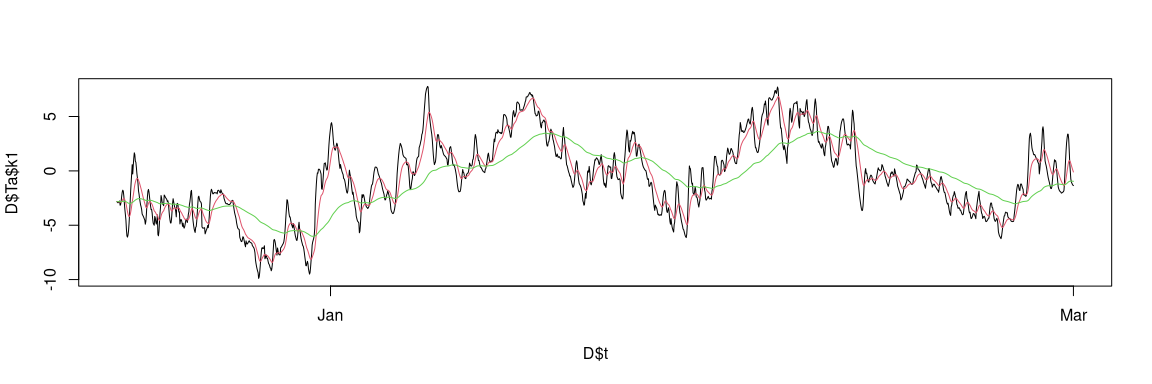hence with a low-pass coefficient a1=0.99, which is very high (max is 1), the Ta forecast is really smoothed, which models a system with a time constant (i.e. slow dynamics, e.g. well insulated and building with lots of concrete).

There are quite a few functions available for input transformations:

• one() generates an matrix of ones (for including an intercept).
• fs() generate Fourier series for modelling harmonic functions.
• bspline() wraps the bs() function for generating base splines.
• pbspline() wraps the pbs() function for generating periodic base splines.
• AR() generates auto-regressive model inputs.

and they can even be combined, see more details in onlineforecasting and in their help description, e.g. ?fs.

Tuning the two parameters: the low-pass filter coefficient a1 and the forgetting factor lambda, can now be done:

# Optimize the parameters
model$prm <- rls_optim(model, D)$par
##     ----------------
##     Ta__a1 lambda
##       0.90   0.99
##        k3   k18   sum
##     0.823 0.817 1.640
##     ----------------
##     Ta__a1 lambda
##      0.901  0.990
##        k3   k18   sum
##     0.823 0.817 1.640
##     ----------------
##     Ta__a1 lambda
##      0.899  0.990
##        k3   k18   sum
##     0.823 0.817 1.639

## ...output cropped

Plot the forecasts (Yhat adheres to the forecast matrix format and in plot_ts the forecasts are lagged k steps to sync with the observations)

# Fit for all horizons
model$kseq <- 1:36 # Fit with RLS fit2 <- rls_fit(model$prm, model, D)
##     ----------------
##     Ta__a1 lambda
##      0.850  0.991
# Take the forecasts
D$Yhat2 <- fit2$Yhat
# Plot all
plot_ts(D, c("^heatload$|^Y"), kseq = c(1,18))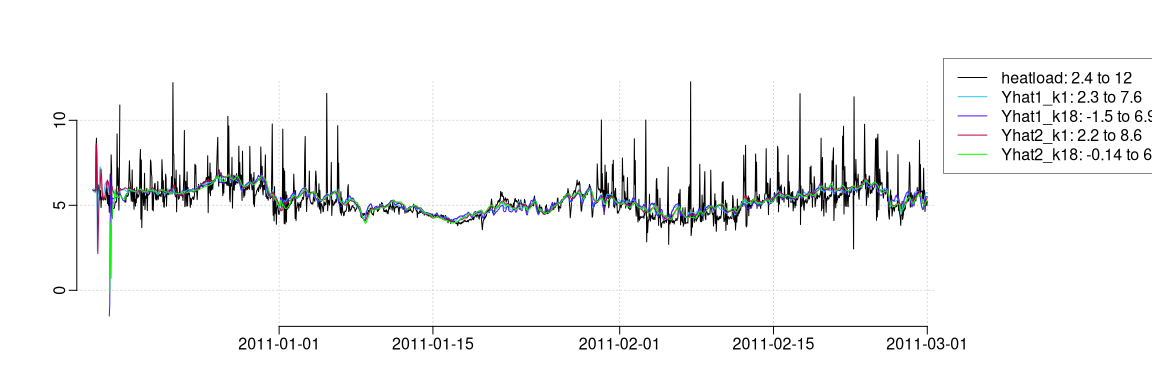We can see the summary: summary(fit2) ## ## Output: heatload ## Inputs: Ta = lp(Ta, a1=0.85) ## mu = one() ## ## Regression parameters: ## lambda = 0.991 ## ## Scoreperiod: 1656 observations are included. ## ## RLS coeffients summary stats (cannot be used for significance tests): ## mean sd min max ## Ta -0.17 0.036 -0.29 0.016 ## mu 5.20 0.180 4.80 6.000 ## ## RMSE: ## k1 k2 k3 k4 k5 k6 k7 k8 k9 k10 k11 k12 k13 k14 k15 k16 ## 0.81 0.82 0.82 0.82 0.82 0.82 0.82 0.82 0.82 0.82 0.82 0.82 0.82 0.82 0.82 0.82 ## k17 k18 k19 k20 k21 k22 k23 k24 k25 k26 k27 k28 k29 k30 k31 k32 ## 0.82 0.82 0.82 0.82 0.82 0.82 0.82 0.82 0.83 0.83 0.83 0.83 0.83 0.83 0.83 0.83 ## k33 k34 k35 k36 ## 0.83 0.83 0.83 0.83 but more interesting is it to see if an improvement was achieved with the low-pass filtering, so calculate the RMSE for both models: # Calculate the score RMSE1 <- summary(fit1, printit=FALSE)$scoreval
RMSE2 <- summary(fit2, printit=FALSE)$scoreval Now, this is calculated for the points included in the scoreperiod, so it’s important to make sure that exactly the same values are forecasted. A check can be done by: # Check that all NAs in the scoreperiod are at the same positions all(is.na(fit1$Yhat[fit1$data$scoreperiod, ]) == is.na(fit2$Yhat[fit2$data$scoreperiod, ])) ##  TRUE Finally, plot the RMSE for the two models: # Plot the score for the two models plot(RMSE1, xlab="Horizon k", ylab="RMSE", type="b", ylim=range(RMSE1,RMSE2)) lines(RMSE2, type="b", col=2) legend("topleft", c("Input: Ta","Input: Low-pass Ta"), lty=1, col=1:2)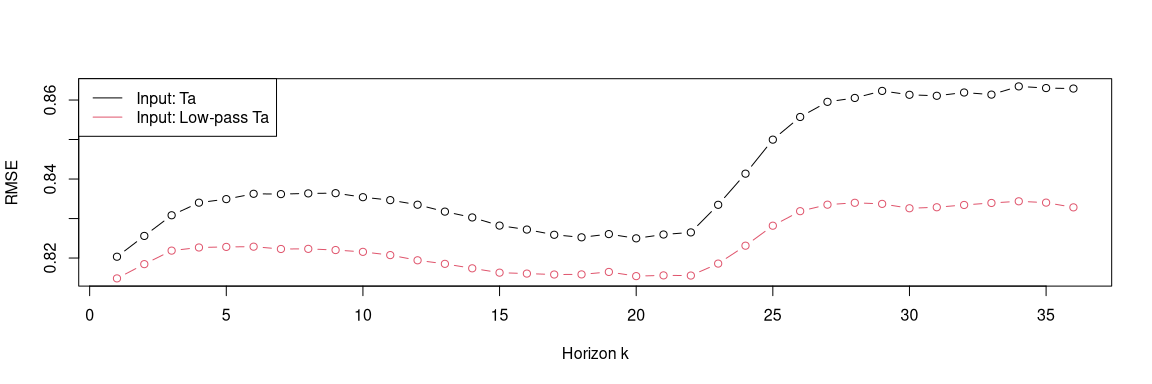We can see, that we obtained improvements. Around 3-4% for the longer horizons. For more on evaluation, see the vignette forecast-evaluation. See more on how to extend this model even further in building heat load forecasting. ## Time of day and using observations as input ### Time of day as input Often we need to have the time of day as an input to a forecastmodel: make_tday(D$t, kseq=1:3)
##          k1 k2 k3
##     1     2  3  4
##     2     3  4  5
##     3     4  5  6
##     4     5  6  7
##     5     6  7  8
##     6     7  8  9
##     7     8  9 10
##     8     9 10 11
##     9    10 11 12
##     10   11 12 13
##     11   12 13 14
##     12   13 14 15
##     13   14 15 16
##     14   15 16 17
##     15   16 17 18
##     16   17 18 19
##     17   18 19 20
##     18   19 20 21
##     19   20 21 22
##     20   21 22 23
##     21   22 23  0
##     22   23  0  1
##     23    0  1  2
##     24    1  2  3
##     25    2  3  4
##     26    3  4  5
##     27    4  5  6

## ...output cropped

So we can use it like this:

D$tday <- make_tday(D$t, 1:36)

See the help ?make_tday for more details.

### Using observations as input

If we want to use observations in inputs to a model, we can use e.g.:

D$Tao <- make_input(D$Taobs, kseq=1:36)
model$add_inputs(Tao = "lp(Tao, a1=0.99)") ## Caching of optimized parameters Working with time consuming calculations caching can be very valuable. The optimization results can be cached by providing a path to a directory, by setting the argument ‘cachedir’ to e.g. “cache”. See the vignette nice-tricks for an example with code. ## Deep clone model Usually, an object of an R6 class can be copied (in memory) deeply with ‘$clone(deep=TRUE)’, however that will result in problems with the forecastmodels, therefore the deep clone must be done by:

m1 <- model\$clone_deep()

See ?R6 for details on R6 objects.